Auencoder是神经网络应用在图像，文本，音频上编码解码的开创性研究，但是Autoencoder在图像编码解码中由于损失函数使用的是像素上的误差， 这就造成了解码重构的图像是迷糊的。AE基础上的改进有VAE，但是VAE依然没有改善生成图像模糊的弊病。GAN引入对抗损失，生成的图像更加清晰。 将GAN的对抗思想应用到Autoencoder中会有什么效果呢？GAIA正是利用这个思想，我们一起来看看。

# 论文引入

Autoencoder在2006由Hinton等人在science提出的，可谓是神经网络应用在图像编码解码的开创之作， AE由编码器Encoder和解码器Decoder组成，编码器将图像或其它高维复杂的数据编码到低维潜在空降，并且通过解码器重构数据。所以说AE是一个双向的过程。 但是AE只是做数据的编码解码，输入数据重构，VAE在AE的基础上将编码得到的潜在空间强制其服从高斯分布，从而实现了数据的生成。同时，VAE的操作 使得潜在空间属于凸集，这就使得潜在向量之间插值做生成是合理的。然而VAE和AE的共病就是生成的图像是模糊的，造成这个原因是由于重构过程中使用的损失函数是在 图像像素上的误差。

GAN的诞生在生成模型上带来了巨大的贡献，GAN由于使用了对抗的思想， 在图像的生成上是sharp的，GAN是直接由先验分布（高斯分布，均匀分布等）采样生成数据，这个过程是直接由$$Z \to X$$是单向过程，由于直接在先验分布上采样 也满足凸集的假设，但是GAN是不可以直接做插值生成的，当然一系列GAN的衍生也慢慢丰富了这条道路。

VAE和GAN在一定成上是优于AE的，但是AE在编码器上由于是直接从原始数据编码得到，所以直接由AE编码得到的潜在向量保留了数据的高维结构特性。 VAE-GAN将VAE和GAN结合了，将图像编码得到的潜在空间作为输入到GAN中做生成，虽然GAN得到了改进，但是训练的稳定性和生成的合理性仍然存在问题。 将AE和GAN结合是GAIA的创新点，直接由图像Encoder得到的潜在向量保留了数据的高维结构特性，但是由于得到的潜在空间是未知的，所以这个潜在空间不一定分从 凸集的特性，GAIA通过将AE应用在生成器和判别器上，同时在损失函数设计上考虑了潜在空间的插值凸集，通过训练使得潜在空间满足凸集性质从而使得插值生成的图像更加合理。

• 提出了一种基于自动编码器（AE）和生成对抗网络（GAN）的神经网络架构
• 通过对潜在空间插值进行对侧训练来促进凸潜在分布
• 使用AE作为GAN的生成器和鉴别器，产生与原始图像的高级和低级特征匹配的非模糊样本
• 可实现插值下图像合理生成

# GAIA模型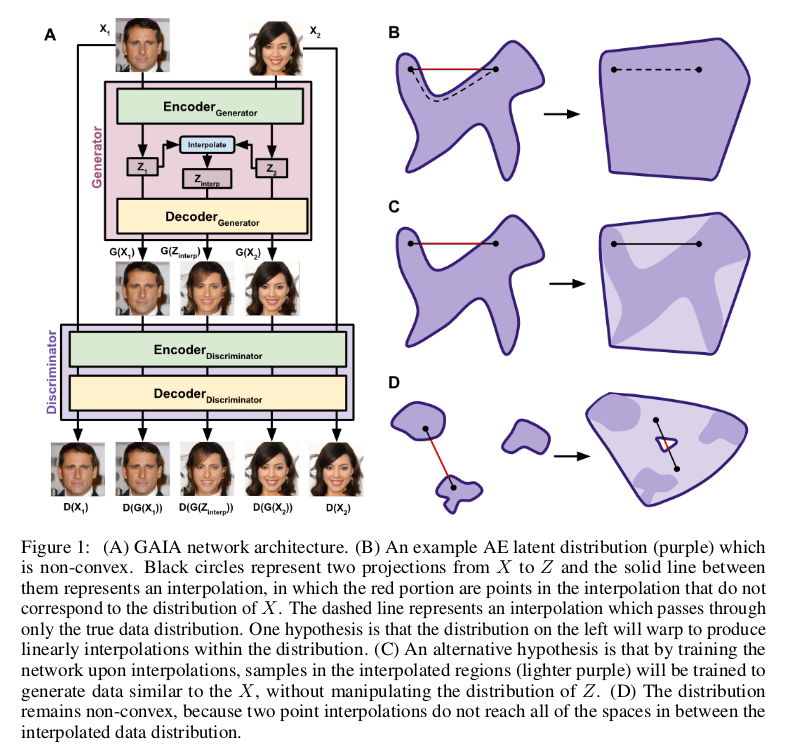# GAIA损失函数

$Z_{interp} = interpolate(Z_{gen}^1,Z_{gen}^2)$

$\Vert X - D(G(X)) \Vert_1 + \Vert G(Z_{interp}) - D(G(Z_{interp})) \Vert_1$

$\Vert X - D(X) \Vert_1 - \Vert X - D(G(X)) \Vert_1 - \Vert G(Z_{interp}) - D(G(Z_{interp})) \Vert_1$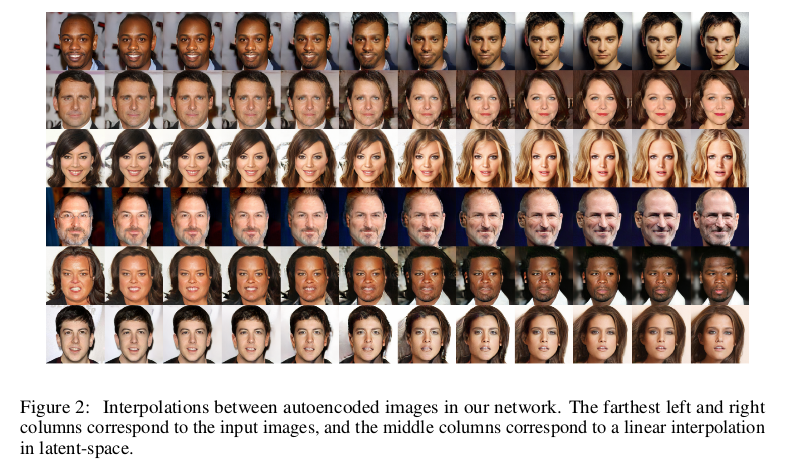# GAIA实验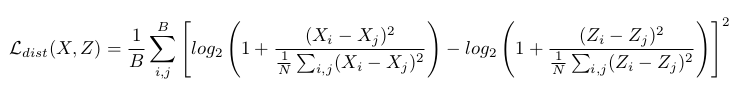$sigmoid(d) = \frac{1}{1+e^{-d*b}}$

$\delta_{Disc} \gets sigmoid(\Vert X - D(X) \Vert_1 - (\Vert X - D(G(X)) \Vert_1 + \Vert G(Z_{interp}) - D(G(Z_{interp})) \Vert_1)/2)$

$\delta_{Gen} \gets 1 - \delta_{Disc}$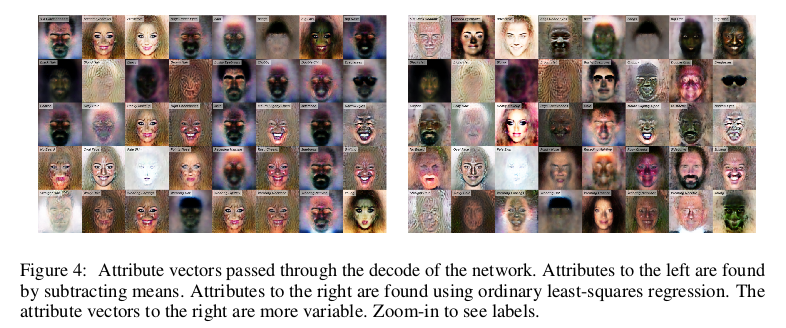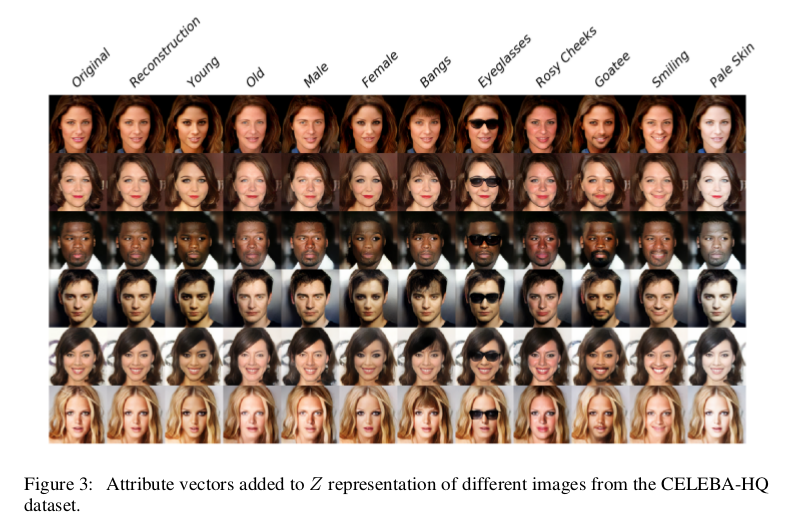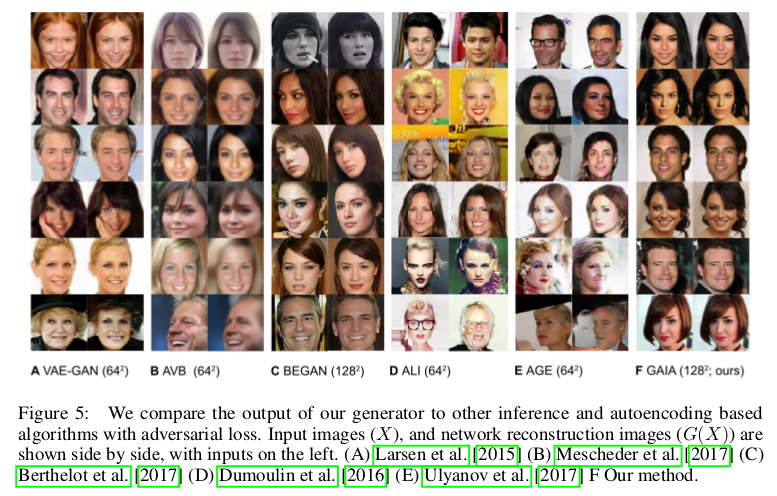# 总结

GAIA中生成器和判别器都采用Autoencoder结构设计，这样保证了数据经过Encoder得到的潜在空间的高维特性，在损失函数上通过生成器和判别器最大最小像素误差达到对抗， 从而实现生成的图像清晰，文中对潜在向量间插值采用训练优化，生成的结果也很合理。